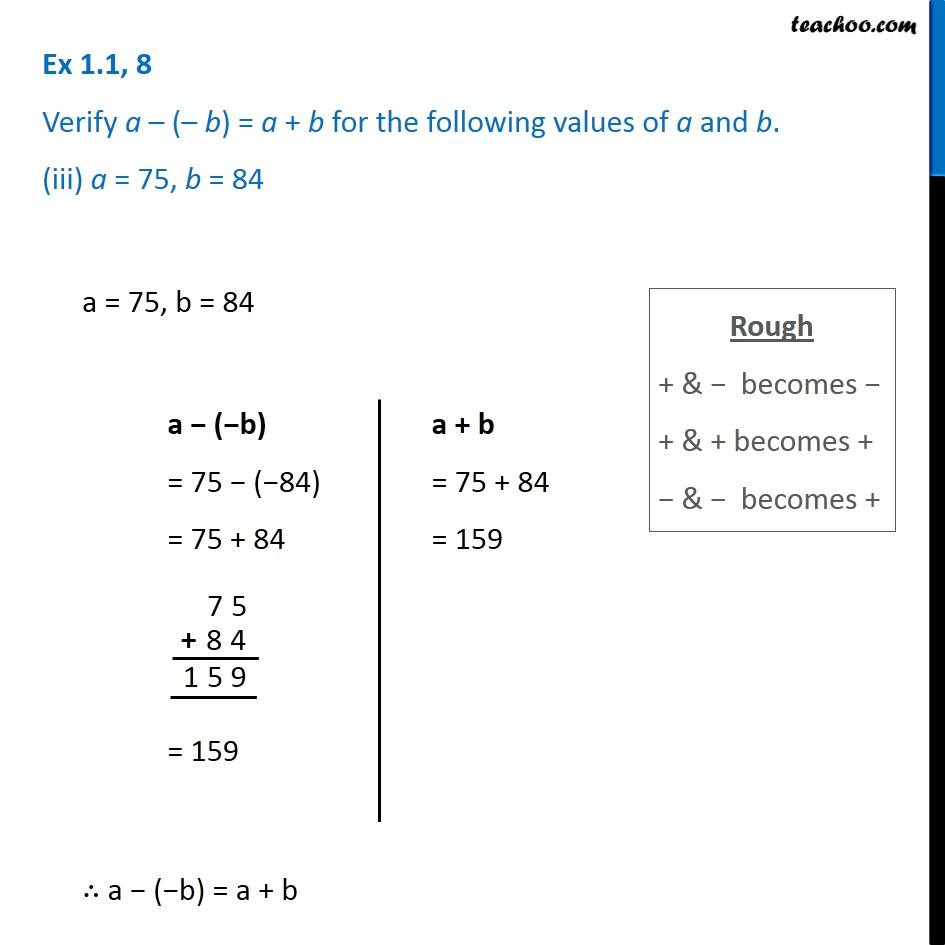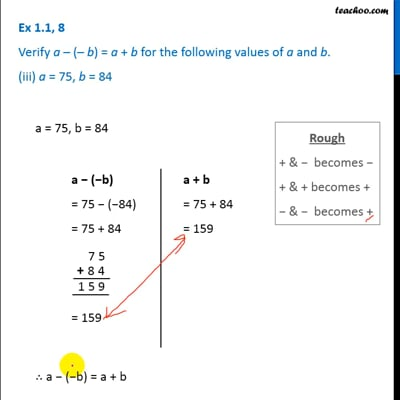Ex 1.1

Chapter 1 Class 7 Integers
Serial order wiseThis video is only available for Teachoo black users

### Transcript

Ex 1.1, 8 Verify a – (– b) = a + b for the following values of a and b. (iii) a = 75, b = 84 Rough + & − becomes − + & + becomes + − & − becomes + a = 75, b = 84 a − (−b) = 75 − (−84) = 75 + 84 = 159 a + b = 75 + 84 = 159 ∴ a − (−b) = a + b